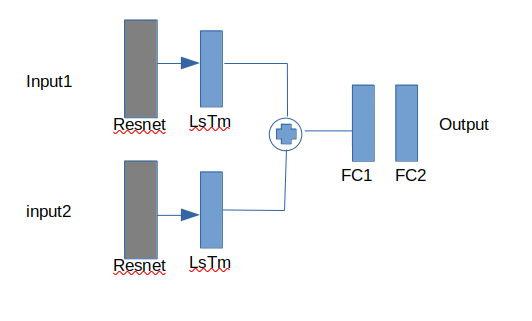# Two stream convLstm

Hello everyone
I was building this architecture and wanted to know if it is the right way to build it. Can you Guys check it out for me? Here are the architecture and the Code.

Architecture:Source Code:

1. First Stream
``````import torch
import torch.nn as nn
import torch.nn.functional as F
from torchvision.models import resnet18, resnet34, resnet50, resnet101, resnet152

# this encoder only supports resnet
class Encoder(nn.Module):
def __init__(self, backbone_name:str):
super(Encoder, self).__init__()
# select a model
if backbone_name == "resnet18":
resnet = resnet18(pretrained=True)
elif backbone_name == "resnet34":
resnet = resnet34(pretrained=True)
elif backbone_name == "resnet50":
resnet = resnet50(pretrained=True)
elif backbone_name == "resnet101":
resnet = resnet101(pretrained=True)
elif backbone_name == "resnet152":
resnet = resnet152(pretrained=True)
else:
assert False, f"'{backbone_name}' backbone is not supported"

self.out_features = resnet.fc.in_features

# remove a fully connected layer
self.encoder = nn.Sequential(*list(resnet.children())[:-2])

# freeze all updatable weights of the encoder
self._freeze_all(self.encoder)

def _freeze_all(self, model:nn.Module):
for param in model.parameters():

def forward(self, x):
x = self.encoder(x)
return x

# this convlstm only supports lstm
class Stream1(nn.Module):
def __init__(self, backbone_name:str, num_classes:int, hidden_size:int = 1024, num_layers:int = 1, bidirectional:bool = True):
super(Stream1, self).__init__()
# freeze
self.encoder = Encoder(backbone_name)
# updateable
self.lstm = nn.LSTM(self.encoder.out_features, hidden_size, num_layers, batch_first=True, bidirectional=bidirectional)

def forward(self, x):
# get shape
print(x.shape)
x = self.encoder(x)
print(x.shape)
b, c, h, w = x.shape
# get (temporal)feature of frames
x = x.view(b, c, h*w)
print(x.shape)
x = x.permute(0, 2, 1)
print(x.shape)
#x = self.lstm(x)[:, -1]
x = self.lstm(x)
return x
``````
1. Second Stream
``````import torch
import torch.nn as nn
import torch.nn.functional as F
from torchvision.models import resnet18, resnet34, resnet50, resnet101, resnet152

# this encoder only supports resnet
class Encoder(nn.Module):
def __init__(self, backbone_name:str):
super(Encoder, self).__init__()
# select a model
if backbone_name == "resnet18":
resnet = resnet18(pretrained=True)
elif backbone_name == "resnet34":
resnet = resnet34(pretrained=True)
elif backbone_name == "resnet50":
resnet = resnet50(pretrained=True)
elif backbone_name == "resnet101":
resnet = resnet101(pretrained=True)
elif backbone_name == "resnet152":
resnet = resnet152(pretrained=True)
else:
assert False, f"'{backbone_name}' backbone is not supported"

self.out_features = resnet.fc.in_features

# remove a fully connected layer
self.encoder = nn.Sequential(*list(resnet.children())[:-2])

# freeze all updatable weights of the encoder
self._freeze_all(self.encoder)

def _freeze_all(self, model:nn.Module):
for param in model.parameters():

def forward(self, x):
x = self.encoder(x)
return x

# this convlstm only supports lstm
class Stream2(nn.Module):
def __init__(self, backbone_name:str, num_classes:int, hidden_size:int = 1024, num_layers:int = 1, bidirectional:bool = True):
super(Stream2, self).__init__()
# freeze
self.encoder = Encoder(backbone_name)
# updateable
self.lstm = nn.LSTM(self.encoder.out_features, hidden_size, num_layers, batch_first=True, bidirectional=bidirectional)

def forward(self, x):
# get shape
print(x.shape)
x = self.encoder(x)
print(x.shape)
b, c, h, w = x.shape
# get (temporal)feature of frames
x = x.view(b, c, h*w)
print(x.shape)
x = x.permute(0, 2, 1)
print(x.shape)
x = self.lstm(x)

return x

``````
1. Ensemble
``````import torch
import torch.nn as nn
import torch.nn.functional as F
from torchvision.models import resnet18, resnet34, resnet50, resnet101, resnet152
from Stream1 import Stream1
from Stream2 import Stream2
import operator

# this convlstm only supports lstm
class Ensemble(nn.Module):
def __init__(self, num_classes:int):
super(Ensemble, self).__init__()
# freeze
self.stream1 = Stream1( backbone_name="resnet18",
num_classes= num_classes,
hidden_size=1024,
num_layers=1,
bidirectional=True)
self.stream2 = Stream2(backbone_name="resnet18",
num_classes= num_classes,
hidden_size=1024,
num_layers=1,
bidirectional=True)
# updateable
self.classifier = nn.Sequential(
nn.Linear(2048,1024),
nn.ReLU(),
nn.Linear(1024, num_classes),
)

def forward(self, x1, x2):
x1 = self.stream1(x1)
x2 = self.stream2(x2)
x3 = tuple(map(operator.add, x1, x2))[:, -1]
print(x3.shape)
x3 = self.classifier(x3)

return x3

if __name__ == "__main__":
x1 = Variable(torch.randn(1, 3, 224, 224))
x2 = Variable(torch.randn(1, 3, 224, 224))
model = Ensemble(120)
output = model(x1,x2)

``````